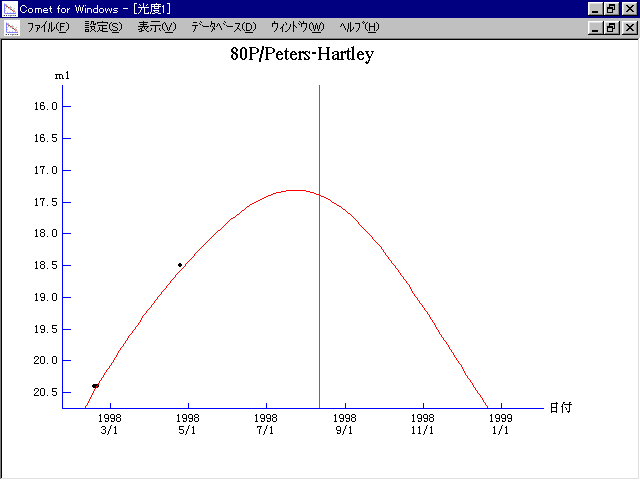# \$B%T!<%?!<%:(B-\$B%O!<%H%l!

80P/Peters-Hartley (1998)###\$B50F;MWAG(B

```   The following improved orbital elements by Kenji Muraoka,
are from 34 observations 1982 to 1998, including 9 Planets,
Moon and 5 minor planets perturbations and non-gravitational
effect of style II.  The mean residual is +/- 0.88 arc seconds.

Epoch  =  1998 Aug. 15.0  TT       JDT = 2451040.5
T  =  1998 Aug. 11.64046       +/- 0.00224 (m.e.) TT
Peri. =  338.40486                +/- 0.00125
Node  =  260.00668                +/- 0.00013   (2000.0)
Incl. =   29.85526                +/- 0.00012
q  =    1.6239230              +/- 0.0000046 AU
e  =    0.5980144              +/- 0.0000024
a  =    4.0397542              +/- 0.0000065 AU
n  =    0.12138685             +/- 0.00000029
P  =    8.120                  +/- 0.0000197  years
A1  =   +0.299                  +/- 0.259
A2  =   -0.07463                +/- 0.00125
```

###\$B@1?^(B###\$B8wEYJQ2=(B

```        m1 = 12.0 + 5 log\$B&\$(B + 20.0 log r
```##### \$B50F;MWAG\$OB<2,7r<#;a\$N7W;;\$K\$h\$k\$b\$N\$G\$9!#(B \$B@1?^\$O(B StellaNavigator Ver.2.0 for Windows (\$B%"%9%H%m%"!<%D(B \$BJTCx(B / \$B%"%9%-!<=PHG6I4)(B) \$B\$G:n@.\$7\$?\$b\$N\$G\$9!#(B \$B8wEY%0%i%U\$O(BComet for Windows\$B\$G:n@.\$7\$?\$b\$N\$G\$9!#(B